## 7.3 Heaps

• An efficient realization of a priority queue uses a (nonlinear) data structure called a heap performs both insertions and removals in logarithmic time O(log n).
7.3.1 The Heap Data Structure
• A heap is a binary tree storing keys at its internal nodes and satisfying the following properties:
• Heap-Order: for every internal node v other than the root, key(v) ≥ key(parent(v))
• Complete Binary Tree: let h be the height of the heap
• for i = 0, … , h − 2, there are 2i nodes of depth i
• at depth h − 1, the internal nodes are to the left of the external nodes
• The last node of a heap is the rightmost internal node of depth h − 1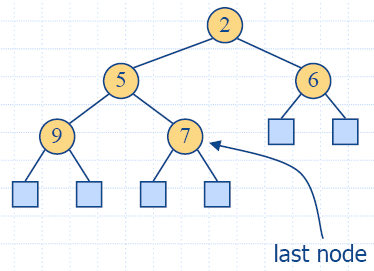Proposition 7.5: A heap T storing n keys has height h = [log(n + 1)].
Proof: (we apply the complete binary tree property)
• Let h be the height of a heap storing n keys
• Since there are 2i keys at depth i = 0, … , h −2 and at least one key at depth h −1, we have n ≥ 1 + 2 + 4 +… + 2h −2 + 1 =  2h −1, thus, n ≥ 2h − 1, i.e. h log n + 1.
• Since for complete binary tree when there are 2h − 1  keys at depth h −1, we have n 1 + 2 + 4 +… + 2h −12h 1, thus, n 2h 1, i.e. h ≤ log (n + 1).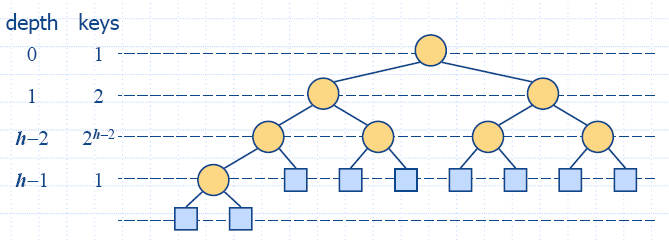7.3.2 Implementing a Priority Queue with a Heap
• We can use a heap to implement a priority queue
• We store a (key, element) item at each internal node
• We keep track of the position of the last node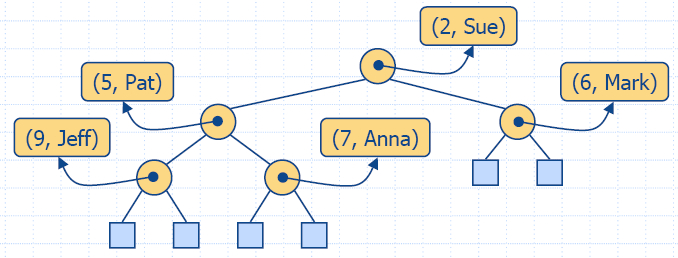The Vector Representation of a Heap
• We can represent a heap with n keys by means of a vector of length n + 1
• For the node at rank i
• the left child is at rank 2i
• the right child is at rank 2i +1
• Links between nodes are not explicitly stored
• The leaves are not represented
• The cell of at rank 0 is not used
• Operation insertItem corresponds to inserting at rank n + 1
• Operation removeMin corresponds to removing at rank 1
• Yields in-place heap-sort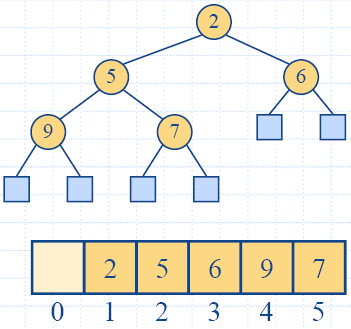Insertion

• Method insertItem of the priority queue ADT corresponds to the insertion of a key k to the heap
• The insertion algorithm consists of three steps
• Find the insertion node z (the new last node)
• Store k at z and expand z into an internal node
• Restore the heap-order property (discussed next)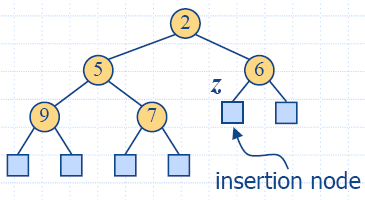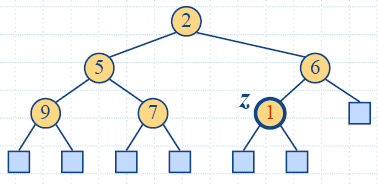Up-Heap Bubbling after an Insertion

• After the insertion of a new key k, the heap-order property may be violated
• Algorithm upheap restores the heap-order property by swapping k along an upward path from the insertion node
• Upheap terminates when the key k reaches the root or a node whose parent has a key smaller than or equal to k
• Since a heap has height O(log n), upheap runs in O(log n) time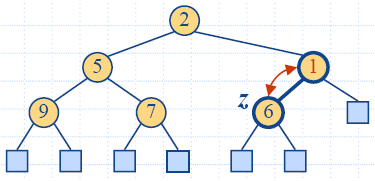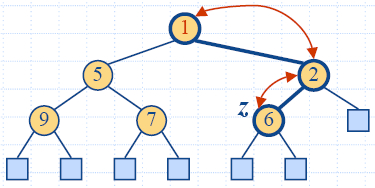Removal
• Method removeMin of the priority queue ADT corresponds to the removal of the root key from the heap
• The removal algorithm consists of three steps
• Replace the root key with the key of the last node w
• Compress w and its children into a leaf
• Restore the heap-order property (discussed next)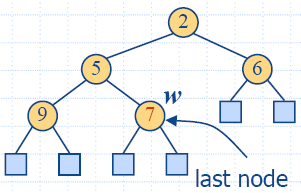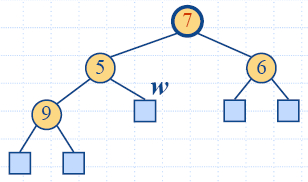Down-Heap Bubbling after a Removal
• After replacing the root key with the key k of the last node, the heap-order property may be violated
• Algorithm downheap restores the heap-order property by swapping key k along a downward path from the root
• Downheap terminates when key k reaches a leaf or a node whose children have keys greater than or equal to k
• Since a heap has height O(log n), downheap runs in O(log n) time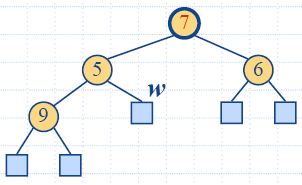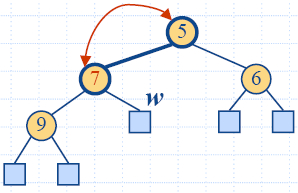Analysis

 Function Time size(), isEmpty() O(1) minElement(), minKey() O(1) insertItem(k,e) O(log n) removeMin() O(log n)

7.3.3 C++ Implementation

html-7.7 (HeapTree)
html-7.8 (HPQ1)
html-7.9 (HPQ2)

VisoAlgo

Heap Visualization

7.3.4 Heap-Sort
• Consider a priority queue with n items implemented by means of a heap
• the space used is O(n)
• methods insertItem and removeMin take O(log n) time
• methods size, isEmpty, minKey, and minElement take O(1) time
• Using a heap-based priority queue, we can sort a sequence of n elements in O(n log n) time
• The resulting algorithm is called heap-sort
• Heap-sort is much faster than quadratic sorting algorithms, such as insertion-sort and selection-sort
Heap Sort